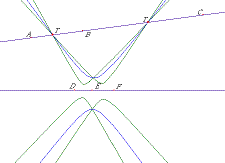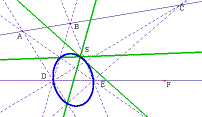## A Family of Conics Associated with the Configuration of Pappus

Leroy J. Dickey

### List of Figures T_o_C

• Figure 1. The conic Q(F).
• Figure 2. The three conics Q(D), Q(E) and Q(F) meet L1 at T1 and T2.
• Figure 3. All six conics: Q(A) Q(B), Q(C), Q(D), Q(E) and Q(F).
• Figure 4. A Steiner conic associated with the configuration of Pappus.

### Abstract T_o_C

In this paper, we show a family of six conics connected with the Configuration of Pappus. These conics meet in interesting ways. There are two points on one of the base lines that are common to three of the conics. There are two points on the other base line that are common to the other three conics. There are two further points that are common to all six conics. These six points together with the intersection of the two base lines form a complete quadrangle. In the second part of this paper, we show the connection between the above six conics and 12 Steiner conics that are connected with the configuration of Pappus.

### The construction T_o_C

• The Base Figure
• Let L1 and L2 be two distinct (base) lines.
• Let O be the point of intersection of the two base lines L1 and L2.
• Let A, B and C be three points on the line L1 that are distinct from each other and distinct from O.
• Let D, E and F be three points on the line L2 that are distinct from each other and distinct from O.
• The Six Conics
We construct six conics, Q(A), Q(B) Q(C), Q(D) Q(E) and Q(F) as follows:
• Let S = { A, B, C, D, E, F }.
• For each point X in S, construct a conic Q(X) as follows:
• Let S(X) be the five points in S \ { X }.
• Let S0(X) be the set of three points in S(X) that are on the line (either L1 or L2) not containing X.
• Let S0(X) = S(X) - S1(X).
• Construct the six lines connecting the 3 points in S1(X) to the 2 points in S2(X).
• These six lines meet in eleven distinct points. Five of the eleven are in S. The remaining six points lie on a conic that we call Q(X), for X = A, B, C, D, E, or F.
• ( Figure 1 shows the particular conic Q(F). )

### The Theorem T_o_C

With reference to the Base Figure and the Six Conics, the following properties hold:
• There are two points, T1 and T2 on the line L1 that the lie on all three conics Q(D), Q(E) and Q(F).
• There are two points, U1 and U2 on the line L2 that lie on all three conics Q(A), Q(B) and Q(C).
• There are two points, V1 and V2, that are diagonal points of the complete quadrangle T1, T2, U1, U2 and lie on all six conics.

### The outline of the proof T_o_C

• Set up the coordinates for the Base Figure.
• Find the equations of the Six Conics Q(A), Q(B), Q(C), Q(D), Q(E) and Q(F).
• Find the coordinates of T1 and T2, the two points of intersection of L1 with Q(F).
• Verify that points T1 and T2 are on the conic Q(D).
• Verify that points T1 and T2 are on the conic Q(E).
• ( Figure 2 shows three conics Q(D), Q(E) and Q(F) and how they meet at the two points T1 and T2 on L1. )

####Figure 2: The three conics Q(D), Q(E) and Q(F) meet L1 at T1 and T2. More about Figure 2.List of Figures

• Find the coordinates of U1 and U2, the two points of intersection of L2 with the conic Q(C).
• Verify that points U1 and U2 are on the conic Q(A).
• Verify that points U1 and U2 are on the conic Q(B).
• Let V1 be the point of intersection of lines T1 U1 and T2 U2
• Let V2 be the point of intersection of lines T1 U2 and T2 U1
• Verify that both V1 and V2 are on all Six Conics.
• ( Figure 3 shows the Six Conics and how they meet by threes at T1, T2 and U1, U2, and how they all meet at V1 and V2. )

### The details of the proof T_o_C

The proof of the theorem is analytic and uses the symbolic algebra programming language MAPLE V.
• The data (source)
This file contains the Maple V source code giving the coordinates for the six points of the base figure. All other calculations are based on these six points.
• The functions (source)
This file contains the Maple V source code for seventeen functions that are used to construct all the other objects of this construction, whether they be points, lines or conics.
• The defined objects
• The Maple V source code for the constructions.
• The new objects consist of the equations of conics and coordinates of new lines and points.
• The proof (validation of incidences).
• The Maple V source code for the validations.
• The results.
The results of the validation section. All claimed incidences are as they should be.

### The Pappus configuration T_o_C

Given any six distinct points points X, Y, Z, U, V, W for which X, Y and Z are on one line and U, V and W are on the another, and all six are distinct from the intersection point of the two lines, the three points YW.ZV, ZU.XW and XV.YU lie on a line denoted by P(X,Y,Z; U,V,W).

### The Steiner points T_o_C

• Se, the "even" Steiner point.
• The three Pappus lines
• P(A,B,C; D,E,F),
• P(A,B,C; E,F,D) and
• P(A,B,C; F,D,E)
coincide at a point denoted by Se.
• The subscript "e" is used because the ordered sequences (D,E,F), (F,D,E) and (E,F,D) are the three even permutations of the three symbols D, E and F.
• So, the "odd" Steiner point.
• The three Pappus lines
• P(A,B,C; F,E,D),
• P(A,B,C; D,F,E) and
• P(A,B,C; E,D,F)
coincide at a point denoted by So.
• The subscript "o" is used because the ordered sequences (F,E,D), (D,F,E) and (E,D,F) are the three odd permutations of the three symbols D, E and F.

### Twelve Steiner conics T_o_C

Both of the points Se and So depend on all six points in S = {A, B, C, D, E, F}. For X in S, the locus of Se, as a function of X, is called Qe(X). Similarly, the locus of So, as a function of X, is called Qo(X). We outline here the proof that Qe(X) and Qe(X) are conics.

For simplicity, we deal specifically with the particular choice X=F, and the other cases for A, B, C, D and E are similar.

• Consider F as a control point (parameter) allowed to move along on the entire line L2.
• The Pappus line P(A,B,C; D,E,F) contains the three points:
• BF.CE
• CD.AF
• AE.BD
• Two of these points depend on F but AE.BD does not. Hence P(A,B,C; D,E,F) turns on AE.BD.
• There is a location of F on the line L2 for which Se coincides with AE.BD, namely the point where L2 intersects the line that joins C and AE.BD.
• Hence the locus of Se(F) includes the point AE.BD.
• (summary) The pappus line P(A,B,C; D,E,F) turns on the point AE.BD. (also contains BF.CE and CD.AF ) (F = L2 . C (AE.BD) implies So = AE.BD.)

----=x=O=x=---- ----=x=O=x=---- ----=x=O=x=----

• The Pappus line P(A,B,C; E,F,D) contains the three points:
• BD.CF
• AF.BE
• Two of these points depend on F but , CE.AD, does not.
• There is a location of F on the line L2 for which Se coincides with CE.AD, namely the point where L2 intersects the line that joins C and BD.AE.
• Hence the locus of Se(F)includes the point BD.AE.
• the third point, CE.AD, is independent of F. Two of the three points depend on F and move as a function of F. turns on the point CE.AD, that is independent of F.
• There is one location of F, on L2, namely the point where L2 intersects the line which joins C and BD.AE, for which Se is BD.AE. Hence the locus of Se(F)includes the point BD.AE.
• In summary, the pappus line P(A,B,C; D,E,F) turns on the point AE.BD. (also contains BF.CE and CD.AF ) (F = L2 . C (AE.BD) implies So = AE.BD.)
• The pappus line P(A,B,C; F,D,E) turns on the point BE.CD. (also contains AD.BF AND AE.CF) (F = L2 . A (BE.CD) implies So = BE.CD )
• The pappus line P(A,B,C; E,F,D) turns on the point CE.AD. (also contains AF.BE and BD.CF) (F = L2 . B (CE.AD) implies So = CE.AD )
• Notable: The three lines
• A(BE.CD),
• C(AE.BD)
coincide. Moreover, if F = L1 . L2, then Se is at this point of coincidence.
• As a function of F, The locus of So, is a conic that contains the five distinct points D, E, AE.BD, BE.CD, CE.AD, no three of which are collinear.
• This conic is denoted by Qo(F) and is defined by the five points D, E, AE.BD, BE.CD, CE.AD .
• Figure 4 shows this conic and three Pappus lines meeting at the Steiner point S0.

####Figure 4. A Steiner conic Se(F) associated with the configuration of Pappus. More about Figure 4.List of Figures

• Similarly, the three Pappus lines P(A,B,C; D,F,E), P(A,B,C; F,E,D) and P(A,B,C; E,D,F) meet at a second Steiner point, S1, whose locus, as a function of F, is a second conic that contains the five points D, E, AD.BE, BD.CE, CD.AE.
• This locus is the conic defined by the five points D, E, AD.BE, BD.CE, CD.AE .
• Take the union of the two sets of five points discovered above,
{ D, E, AE.BD, BE.CD, CE.AD } and
{ D, E, AD.BE, BD.CE, CD.AE }
and remove the two points of S2(F), (namely D and E), to obtain the set of six points { AE.BD, BE.CD, CE.AD, AD.BE, BD.CE, CD.AE }. All six of these points lie on the conic Q(F). [Pascal]

### The Connection between Steiner Conics and Q(A), Q(B), Q(C), Q(D), Q(E), Q(F) T_o_C

. There is a connection between the six conics Q(A), Q(B), Q(C), Q(D), Q(E) and Q(F) (defined above),
• In the same way, for any X in the set S, the loci of the two Steiner points S0 and S1, as a function of X, (the conics Q0(X) and Q1(X), respectively) lead us to six interesting points, any five of which determine / characterize the conic Q(X). This brings us full circle back to the beginning of the paper.

The end.